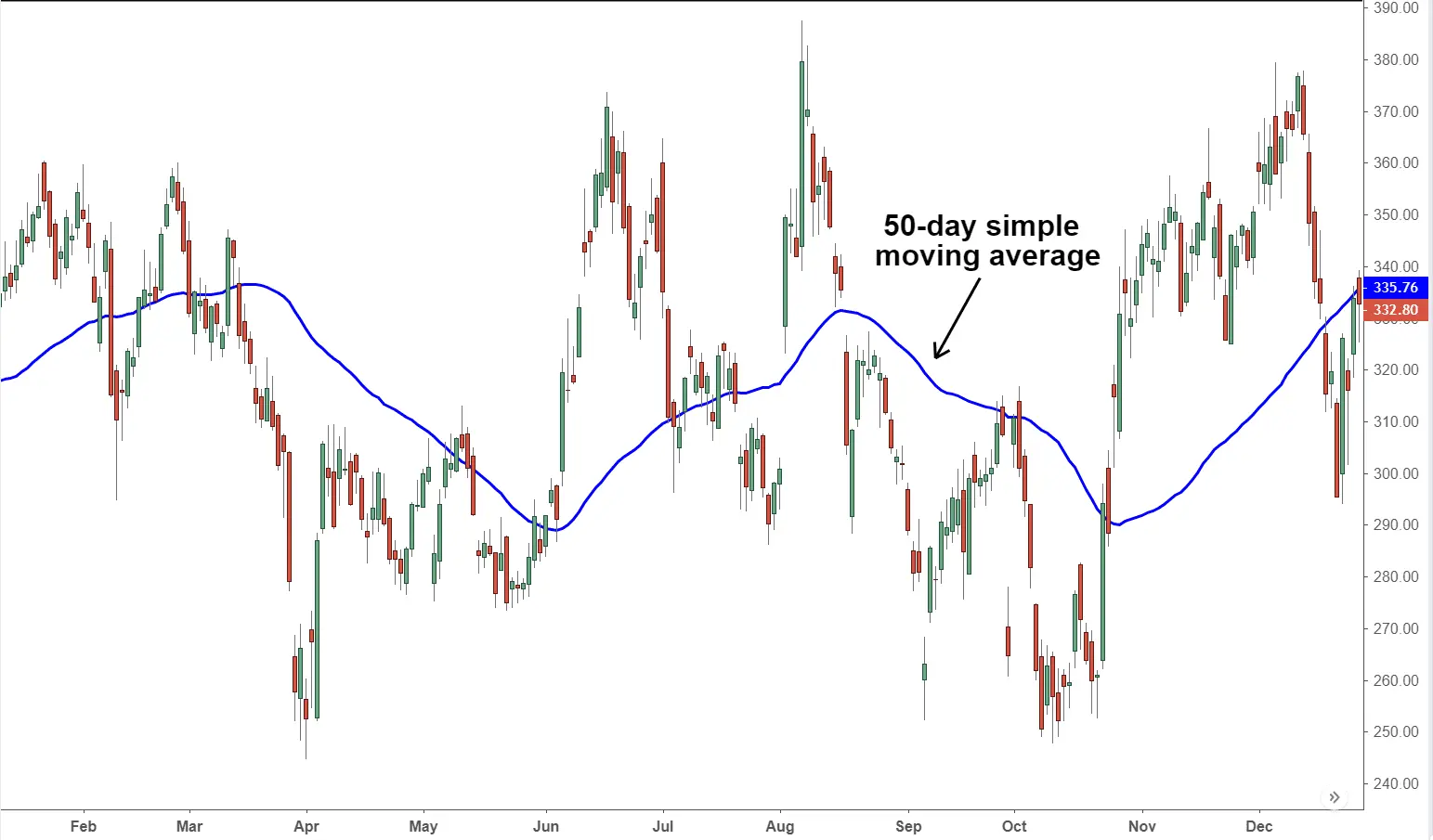# Moving Average for NumPy Array in Python

Manav Narula Oct 10, 2023

Moving average is frequently used in studying time-series data by calculating the mean of the data at specific intervals. It is used to smooth out some short-term fluctuations and study trends in the data. Simple Moving Averages are highly used while studying trends in stock prices.

Weighted moving average puts more emphasis on the recent data than the older data.

The graph below will give a better understanding of Moving Averages.In this tutorial, we will discuss how to implement moving average for numpy arrays in Python.

## Use the `numpy.convolve` Method to Calculate the Moving Average for NumPy Arrays

The `convolve()` function is used in signal processing and can return the linear convolution of two arrays. What is being done at each step is to take the inner product between the array of ones and the current window and take their sum.

The following code implements this in a user-defined function.

``````import numpy as np

def moving_average(x, w):
return np.convolve(x, np.ones(w), "valid") / w

data = np.array([10, 5, 8, 9, 15, 22, 26, 11, 15, 16, 18, 7])

print(moving_average(data, 4))
``````

Output:

``````[ 8.    9.25 13.5  18.   18.5  18.5  17.   15.   14.  ]
``````

## Use the `scipy.convolve` Method to Calculate the Moving Average for NumPy Arrays

We can also use the `scipy.convolve()` function in the same way. It is assumed to be a little faster. Another way of calculating the moving average using the numpy module is with the `cumsum()` function. It calculates the cumulative sum of the array. This is a very straightforward non-weighted method to calculate the Moving Average.

The following code returns the Moving Average using this function.

``````def moving_average(a, n):
ret = np.cumsum(a, dtype=float)
ret[n:] = ret[n:] - ret[:-n]
return ret[n - 1 :] / n

data = np.array([10, 5, 8, 9, 15, 22, 26, 11, 15, 16, 18, 7])

print(moving_average(data, 4))
``````

Output:

``````[ 8.    9.25 13.5  18.   18.5  18.5  17.   15.   14.  ]
``````

## Use the `bottleneck` Module to Calculate the Moving Average

The `bottleneck` module is a compilation of quick numpy methods. This module has the `move_mean()` function, which can return the Moving Average of some data.

For example,

``````import bottleneck as bn
import numpy as np

def rollavg_bottlneck(a, n):
return bn.move_mean(a, window=n, min_count=None)

data = np.array([10, 5, 8, 9, 15, 22, 26, 11, 15, 16, 18, 7])

print(rollavg_bottlneck(data, 4))
``````

Output:

``````[  nan   nan   nan  8.    9.25 13.5  18.   18.5  18.5  17.   15.   14.  ]
``````

Since the time window interval is 4, there are three nan values at the start because the Moving Average could not be calculated for them.

## Use the `pandas` Module to Calculate the Moving Average

Time series data is mostly associated with a `pandas` DataFrame. Therefore the library is well equipped for performing different computations on such data.

We can calculate the Moving Average of a time series data using the `rolling()` and `mean()` functions as shown below.

``````import pandas as pd
import numpy as np

data = np.array([10, 5, 8, 9, 15, 22, 26, 11, 15, 16, 18, 7])

d = pd.Series(data)

print(d.rolling(4).mean())
``````

Output:

``````0       NaN
1       NaN
2       NaN
3      8.00
4      9.25
5     13.50
6     18.00
7     18.50
8     18.50
9     17.00
10    15.00
11    14.00
dtype: float64
``````

We first convert the numpy array to a time-series object and then use the `rolling()` function to perform the calculation on the rolling window and calculate the Moving Average using the `mean()` function.

Here also since, the time window interval is 4, there are three nan values at the start because the moving average could not be calculated for them.

Author: Manav Narula

Manav is a IT Professional who has a lot of experience as a core developer in many live projects. He is an avid learner who enjoys learning new things and sharing his findings whenever possible.